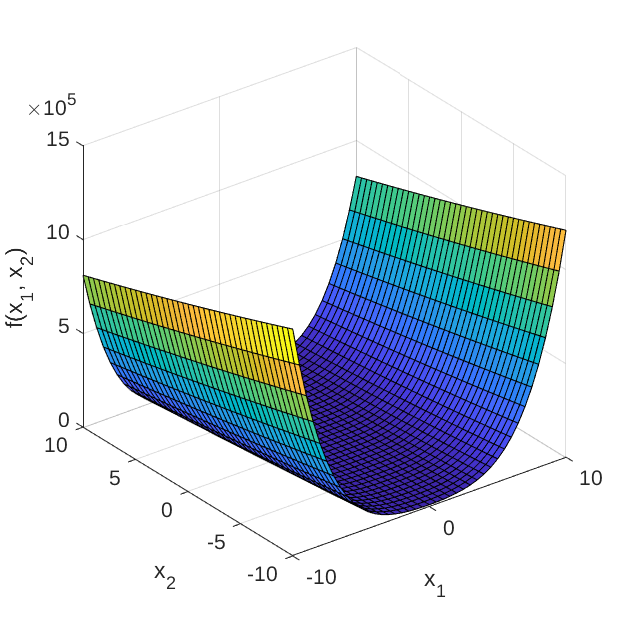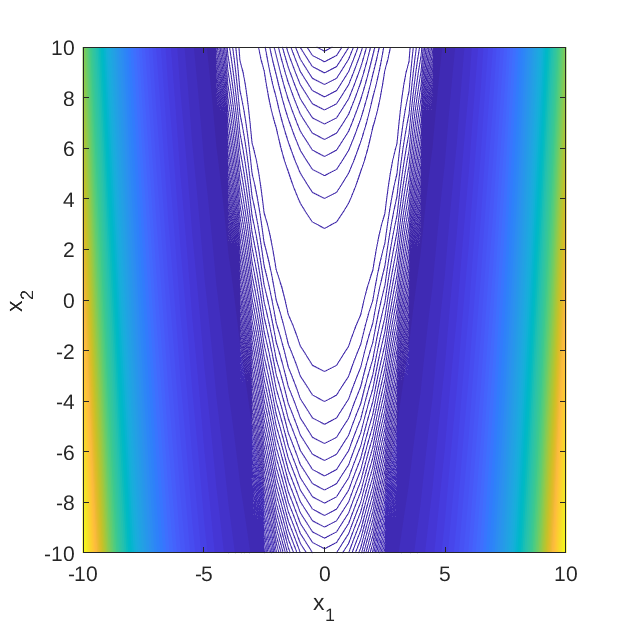# Rosenbrock's Function

The Rosenbrock’s function, also known as the valey or banana function, is commonly used as a test function for optimization algorithms, particularly for gradient-based algorithms since convergence towards its optimum is rather challenging (Forrester et al., 2008; Picheny et al., 2012).

## Description

The analytic expression of the Rosenbrock’s function is given as:

f(\mathbf{x}) = \sum_{m=1}^{M-1}\left[ 100 (x_{m+1} - x_m^2)^2 + (x_m - 1)^2 \right],

where \mathbf{x} = \{x_m,\}_{m=1}^{M} are input variables; and M is a positive integer.

Figure 1 and 2 show the surface and contour plots of the 2-dimensional Rosenbrock’s function evaluated at \mathbf{x} \in [-10, 10]^2​.Figure 1: Surface plot of the 2-dimensional Rosenbrock’s function.Figure 2: Contour plot of the 2-dimensional Rosenbrock’s function.

## Inputs

The function is typically evaluated on the hypercube \mathbf{x} \in [-5, 10]^M. For computer experiment purposes, the input variables are modeled as independent uniform random variables.

No Variable Distribution Parameters
1 x_1 Uniform x_{1,\min} = -5,
x_{1,\max} = 10
\vdots \vdots \vdots \vdots
M x_M Uniform x_{M,\min} = -5,
x_{M,\max} = 10

## Reference values

The Rosenbrock’s function is a unimodal function and its global minimum, f(\mathbf{x}^*) = 0 is located at \mathbf{x}^* = (1, \ldots, 1).

## Resources

The vectorized implementation of the M-dimensional Rosenbrock’s function in MATLAB as well as the script file with the model and probabilistic inputs definitions for the function in UQLAB can be downloaded below:

uq_rosenbrock.zip (2.2 KB)

The contents of the file are:

Filename Description
uq_rosenbrock.m vectorized implementation of the Rosenbrock’s function
uq_Example_rosenbrock.m definitions for the model and probabilistic inputs in UQLab
LICENSE license for the function (BSD 3-Clause)

## References

• A. Forrester, A. Sóbester, and A. Keane, Engineering Design via Surrogate Modelling: A Practical Guide, West Sussex: John Wiley & Sons Ltd., 2008, pp. 196-197. DOI:10.1002/9780470770801
• V. Picheny, T. Wagner, and D. Ginsbourger, “A benchmark of kriging-based infill criteria for noisy optimization,” HAL, hal-00658212, 2012. URL